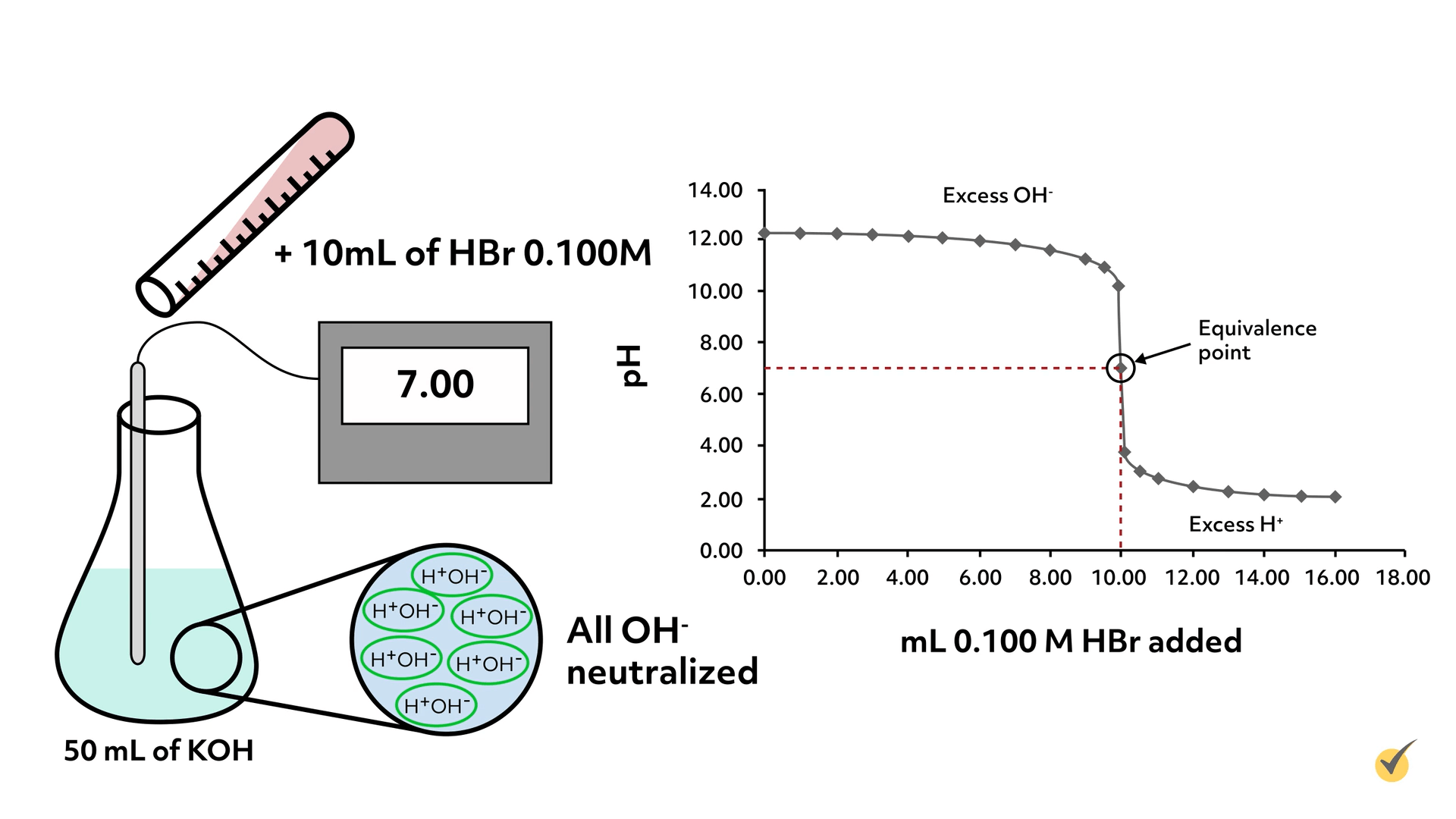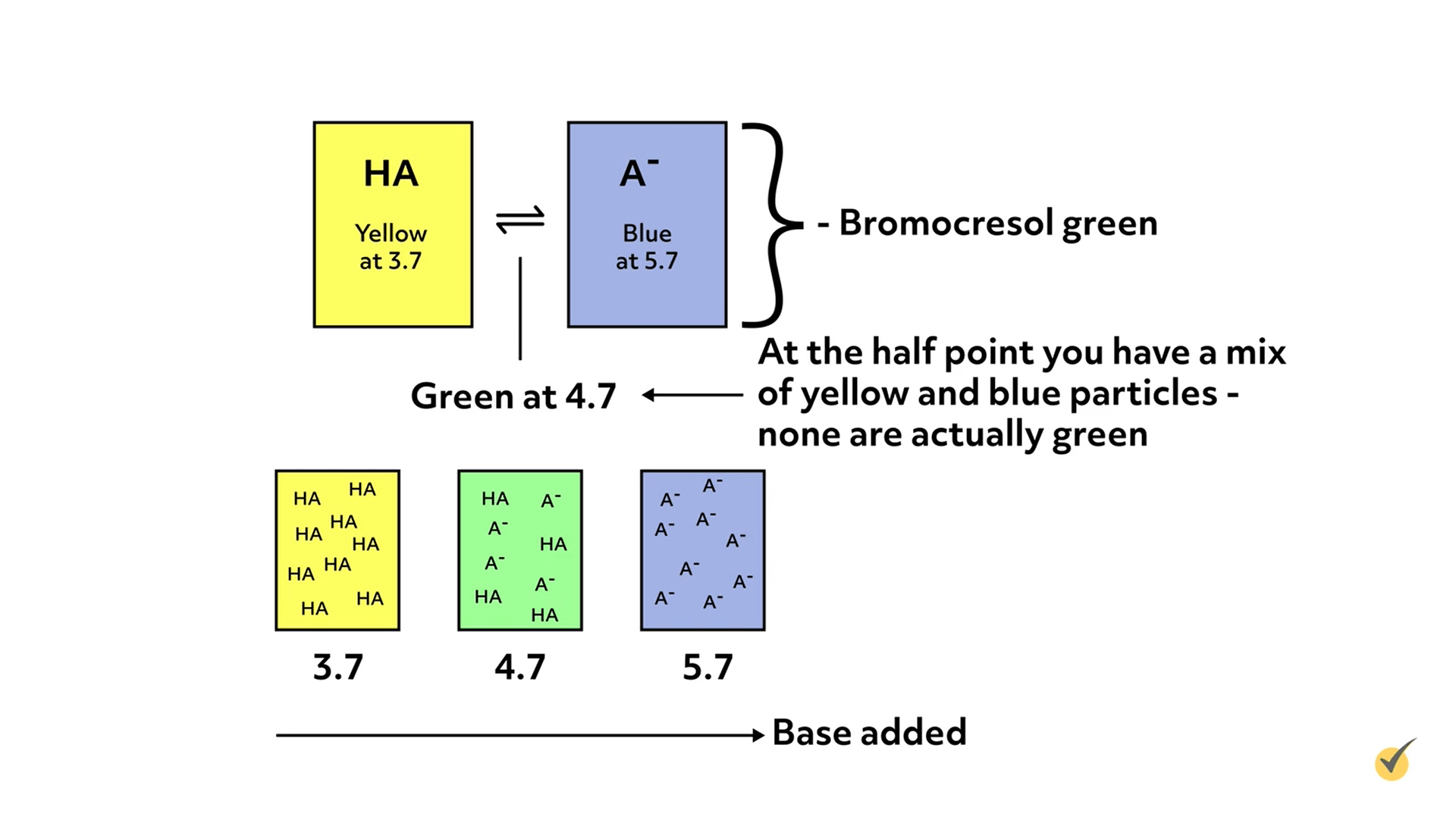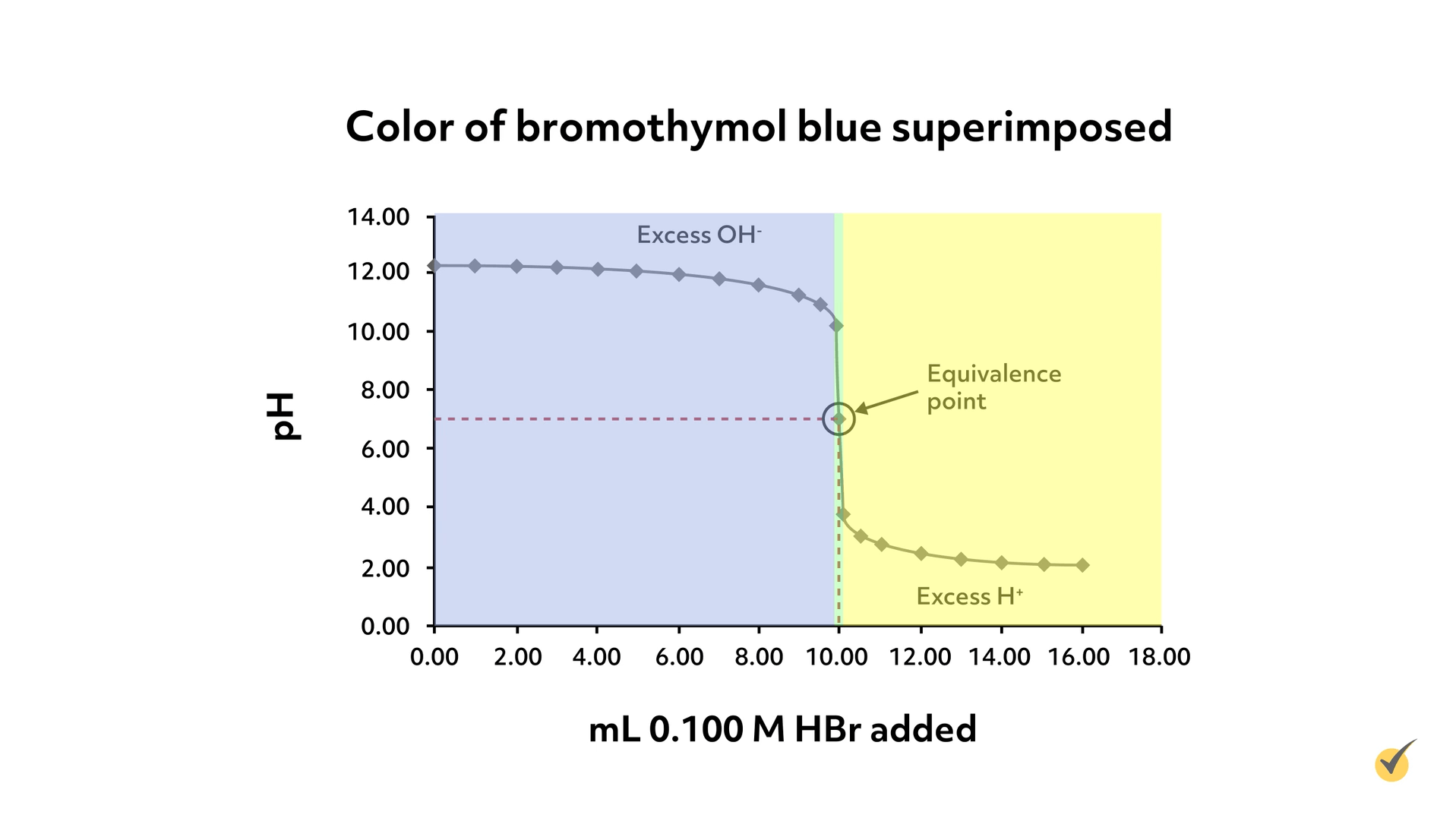# TitrationHi, and welcome to this video on titrations!

Let’s start with a hypothetical. Imagine you walk into a lab and there’s a bottle marked “KOH solution”, but no concentration is listed. You’d like to use it in your lab work, but you have no idea how much KOH (potassium hydroxide) is actually in the solution. What are you going to do?! Simple! A titration!

Titrations are a form of analysis used to determine the concentration of a particular substance in a solution. We refer to the substance being investigated as the ‘analyte.’ Titrations are a common and essential analytical lab technique. The general process is as follows:

1. We have an unknown amount of analyte in solution A.
2. We slowly add solution B to solution A, typically using a burette, which makes measuring small volumes easy. Solution B has been precisely made with a substance that reacts with the analyte in solution A. This substance is called the ‘titrant.’
3. As solution B is added to solution A, we monitor the reaction and watch for an indication that we have reached the equivalence point – where the quantity of titrant is stoichiometrically equivalent to the analyte. What form this cue takes depends on the type of chemistry happening in the titration. It might be a color change, a sudden change in voltage or pH, the formation of a precipitate, or a number of other indicators.
4. Once we know the volume of solution B needed to react with solution A, we can calculate how much analyte is in solution A.

Now that we have the general idea of how titrations work, let’s consider the specific chemistry that takes place. Today, we’ll look at an example of acid-base titration but there are many more varieties.

Let’s return to our hypothetical scenario of a bottle of unknown KOH solution. We’re going to determine the concentration of KOH by titrating with a solution of hydrogen bromide.

To start, we need to set up the balanced chemical reaction. In the general process we outlined earlier, we assumed that the analyte and titrant were stoichiometrically equivalent; however, this is not always the case, and we should always start a titration by understanding the actual chemistry that is taking place.

In this case, the reaction between KOH and HBr is fairly simple and the stoichiometric ratio is one to one.

$$KOH\left ( aq \right )+HBr\rightarrow H_{2}O+K^{+}\left ( aq \right )+Br^{-}\left ( aq \right )$$

or just

$$H^{+}+OH^{-}\rightarrow H_{2}O$$
$$K=10^{14}$$

Because the equilibrium constant, K, is so large for this reaction, we can confidently say that this reaction “goes to completion”. In other words, when we add HBr solution to the KOH solution, any hydrogen ion will immediately react with a hydroxide ion.

To track the progress of this titration, we will monitor the pH of the solution. When enough hydrogen bromide has been added to react with the potassium hydroxide, the pH will equal 7 because all of the hydroxide ions will have been neutralized. In other words, the pH equals 7 at the equivalence point.

So let’s begin the titration. Our hydrogen bromide solution was carefully made and has a concentration of $$0.100\text{ M}$$ (moles per liter). We begin by pouring out $$50\text{ mL}$$ of the potassium hydroxide solution and taking its pH with a calibrated meter:

Then we begin to add small increments of the hydrogen bromide solution and continue to track the pH.

Here is a table and graph showing the results of the titration.This is called a titration curve and is often the form the results display when carrying out titrations.

As you can tell from the titration curve, once we began adding the hydrogen bromide solution, the pH began to drop, but only very slowly. As we got closer to the equivalence point, the drop in pH increased dramatically.

From the graph, we can see that pH 7 was reached when $$10.00\text{ mL}$$ of $$0.100\text{ M}$$ hydrogen bromide solution had been added. For this reaction, that means all of the KOH has been neutralized and thus the number of molecules of KOH is equivalent to the number of molecules of HBr. The equivalence point coincides with the steepest part of the titration curve for all titrations. Using this information, we can now calculate the concentration of the KOH solution.

$$\left ( 10.00\text{ mL} \right )\left ( \frac{1L}{1000\text{ mL}} \right )\left ( \frac{0.100\text{ mol}\text{ HBr}}{1\text{ L}} \right )=0.00100\text{ mol}\text{ HBr}$$

So it took $$0.001$$ moles of HBr to neutralize the KOH in solution, which means there must have been $$0.001\text{ mol}$$ of KOH because it’s 1:1 stoichiometrically. Since we titrated $$50.00\text{ mL}$$ of KOH solution, we can now calculate the concentration to be $$0.0200\text{ M}$$

$$\frac{0.00100\text{ mol}\text{ KOH}}{0.0500\text{ L}}=0.0200\text{ M}\text{ KOH}\text{ solution}$$

Very importantly, note that the quality of our titration was only as good as the certainty in our hydrogen bromide solution. If we had that concentration wrong, then we would have no confidence in our calculated concentration of the potassium hydroxide solution. Consequently, quality titrations are only carried out with primary or secondary standards – these are solutions that are precisely made, pure, and stable over long periods of time.

This was a very simple example of an acid-base titration, in large part because HBr is a strong acid and KOH is a strong base. However, when we’re dealing with weak acids and bases that only partially dissociate in water, the equivalence point is not at a pH of 7. In fact, when titrating a weak acid with a strong base (or vice versa), you’re actually creating a buffer and will need to apply the Henderson-Hasselbalch equation. That is beyond what we will cover today, but it’s important to know that not all acid-base titrations are as straightforward as our example.

Furthermore, many acid-base titrations are not carried out with the help of a pH meter. Instead, an indicator is used to monitor a change in pH over a small range.

Indicators are weak acids or bases that change colors when they are deprotonated or protonated, respectively. They are added in small amounts to the analyte solution, so as not to interfere with the actual titration. As titrant is added and the pH changes, the color of the indicator will gradually change as it either protonates or deprotonates, which gives an estimate of the solution’s pH. The pH at which the color changes for any given indicator is dependent on the pKa or pKb of the indicator, which in turn, depends on the specific properties of the molecule.

For example, bromocresol green changes from yellow to blue over the pH range of 3.7 to 5.7.There are many different indicators that cover a variety of pH ranges, so it is possible to carefully select one for a given acid-base titration such that the color change occurs around the expected equivalence point. Thus, when the indicator changes color, you can mark the endpoint of the titration. The endpoint, as determined by the indicator, is only an approximation of the equivalence point, but it should be close enough if a suitable indicator were selected. And this is true for other types of titrations and indicators.

So, for example, if our pH meter were broken when we were doing the titration of potassium hydroxide solution with hydrogen bromide, we could have used an indicator instead.

To select an appropriate indicator, we could look for one with a color change around a pH of 7. Bromocresol green, our indicator from before, would not work well for this titration because it wouldn’t change color at the equivalence point- it would remain blue until a pH of 5.7. Bromothymol blue, on the other hand, changes from yellow to blue over the range of 6.0-7.6, which, of course, includes 7, so would be an appropriate indicator for this titration.As you can see from the graph, there’s only a very small window where the bromothymol blue would be green and it coincides with where we expect our equivalence point.

Of course, this is more of an approximation than what a chemist would probably be expected to do in an analytical lab, but hopefully it helped you learn the principles of titration.

Now, before we go, let’s go over a couple of review questions:

1. Which of the following best describes a titration?

1. A form of analysis used to determine the concentration of an analyte in solution
2. A form of analysis used to determine the heat released or absorbed in a chemical reaction
3. A form of analysis used to determine the pH levels in a solution.

2. What is a pH indicator?

1. A strong acid or base that changes color only when protonated
2. A weak acid or base that changes color when deprotonated or protonated
3. Any acid or base that changes color when deprotonated or protonated

That’s all for this review! Thanks for watching, and happy studying!